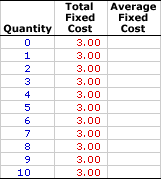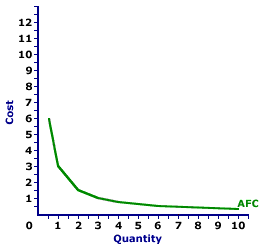Wednesday  May 31, 2023
 AmosWEB means Economics with a Touch of Whimsy!INDUCED CHANGE: A change in aggregate expenditures, especially consumption expenditures, that is "induced" or triggered by a change in national income or gross domestic product. Induced changes form the foundation for the multiplier effect, which is set in motion by autonomous changes in aggregate expenditures. In terms of Keynesian economics and the Keynesian cross diagram, induced changes are seen as a movement along in the aggregate expenditures line. This two step process, autonomous changes causing induced changes, is key to explaining business cycle fluctuations.AVERAGE FIXED COST:

Total fixed cost per unit of output, found by dividing total fixed cost by the quantity of output. When compared with price (per unit revenue), average fixed cost (AFC) indicates whether or not a profit-maximizing firm should shutdown production in the short run. Average fixed cost is one of three average cost concepts important to short-run production analysis. The other two are average total cost and average variable cost. A related concept is marginal cost.
Average fixed cost is the total fixed cost per unit of output incurred when a firm engages in short-run production. It can be found in two ways. Because average fixed cost is total fixed cost per unit of output, it can be found by dividing total fixed cost by the quantity of output. Alternatively, because total fixed cost is the difference between total cost and total variable cost, average fixed cost can be derived by subtracting average variable cost from average total cost.

Average fixed cost decreases with additional production. The logic behind this relation is relatively simple. Because fixed cost is FIXED and does not change with the quantity of output, a given cost is spread more thinly per unit as quantity increases. A thousand dollars of fixed cost averages out to \$10 per unit if only 100 units are produced. But if 10,000 units are produced, then the average shrinks to a mere 10 cents per unit.

Average fixed cost, when combined with price, indicates whether or not a firm should shut down production in the short run. If price is greater than average fixed cost, then the firm is able to pay, at least, fixed cost. Even though it might be incurring an economic loss, it will lose less by producing than by shutting down production. If, however, price is less than average fixed cost, then the firm is better off shutting down production.

### Calculating Average Fixed Cost

The standard method of calculating average fixed cost is to divide total fixed cost by the quantity of output, illustrated by this equation:
 average fixed cost = total fixed costquantity of output
An alternative specification for average fixed cost is found by subtracting average variable cost from average fixed cost:
 average fixed cost = average total cost - average variable cost
An alternative equation computes total fixed cost from average fixed cost:
 total fixed cost = average fixed cost x quantity of output

### Stuffed Animal Cost

Average Fixed CostInsight into average fixed cost can be had using the table to the right, which presents the total fixed cost of producing Wacky Willy Stuffed Amigos. The first column is the quantity of Stuffed Amigos rolling off the assembly line, ranging from 0 to 10. The second column is the total fixed cost of producing each quantity, which is constant at \$3. If 5 Stuffed Amigos are produced, then the total fixed cost incurred in their production is \$3. The production of 9 Stuffed Amigos also incurs a total fixed cost of \$3.

Deriving average fixed cost is as simple as dividing the second column of total fixed cost values by the first column of output quantity values. The average fixed cost of producing 1 Stuffed Amigo is relatively easy--divide \$3 by 1 Stuffed Amigo. If the result of this calculation is not readily obvious, click the [One] button.

The average fixed cost of two Stuffed Amigos might be a little less obvious, but not much. Click the [Two] button to reveal that the average fixed cost is \$1.50, which is \$3 divided by 2 Stuffed Amigos. A click of the [Three] shows that the average fixed cost of producing 3 Stuffed Amigos is \$1, \$3 divided by 3. The remaining average fixed cost values can be displayed by clicking the [Remaining] button.

With all numbers displayed, what interesting insight into average fixed cost can be had?

• First, average fixed cost is high at relatively small output quantities and low at relatively large output quantities. The reason, of course, is that as output increases, a given fixed cost is spread more thinly over a larger quantity.

• Second, average fixed cost remains positive, it never reaches a zero value and never turns negative. The only way for negative average fixed cost is for negative total fixed cost, which makes neither theoretical nor practical sense.

### The Average Fixed Cost Curve

Average Fixed Cost CurveThe relation between average fixed cost and the quantity of production can be represented by a curve, such as the one conveniently presented in the exhibit to the right.

The key feature of this average fixed cost curve is the shape. The average fixed cost curve is negatively sloped. Average fixed cost is relatively high at small quantities of output, then declines as production increases. The more production increases, then the more average fixed cost declines. The reason behind this perpetual decline is that a given FIXED cost is spread over an increasingly larger quantity of output.

 <= AVERAGE FACTOR COST, PERFECT COMPETITION AVERAGE FIXED COST CURVE =>Recommended Citation:

AVERAGE FIXED COST, AmosWEB Encyclonomic WEB*pedia, http://www.AmosWEB.com, AmosWEB LLC, 2000-2023. [Accessed: May 31, 2023].

Check Out These Related Terms...

Or For A Little Background...

And For Further Study...
Search Again?BROWN PRAGMATOX[What's This?] Today, you are likely to spend a great deal of time strolling through a department store hoping to buy either a birthday gift for your uncle or a pair of red and purple designer socks. Be on the lookout for telephone calls from long-lost relatives.Your Complete ScopeThe penny is the only coin minted by the U.S. government in which the "face" on the head looks to the right. All others face left."Always dream and shoot higher than you know how to. Don't bother just to be better than your contemporaries or predecessors. Try to be better than yourself."-- William Faulkner, writerMImportsA PEDestrian's Guide Xtra CreditTell us what you think about AmosWEB. Like what you see? Have suggestions for improvements? Let us know. Click the User Feedback link.| | | | | | | | | | |
| | | |

Thanks for visiting AmosWEB